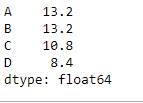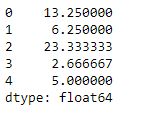# Python | Pandas dataframe.mean()

Python is a great language for doing data analysis, primarily because of the fantastic ecosystem of data-centric python packages. Pandas is one of those packages and makes importing and analyzing data much easier.

Pandas` dataframe.mean()` function return the mean of the values for the requested axis. If the method is applied on a pandas series object, then the method returns a scalar value which is the mean value of all the observations in the dataframe. If the method is applied on a pandas dataframe object, then the method returns a pandas series object which contains the mean of the values over the specified axis.

Syntax: DataFrame.mean(axis=None, skipna=None, level=None, numeric_only=None, **kwargs)

Parameters :
axis : {index (0), columns (1)}
skipna : Exclude NA/null values when computing the result

level : If the axis is a MultiIndex (hierarchical), count along a particular level, collapsing into a Series

numeric_only : Include only float, int, boolean columns. If None, will attempt to use everything, then use only numeric data. Not implemented for Series.

Returns : mean : Series or DataFrame (if level specified)

Example #1: Use `mean()` function to find the mean of all the observations over the index axis.

 `# importing pandas as pd ` `import` `pandas as pd ` ` `  `# Creating the dataframe  ` `df ``=` `pd.DataFrame({``"A"``:[``12``, ``4``, ``5``, ``44``, ``1``], ` `                   ``"B"``:[``5``, ``2``, ``54``, ``3``, ``2``],  ` `                   ``"C"``:[``20``, ``16``, ``7``, ``3``, ``8``], ` `                   ``"D"``:[``14``, ``3``, ``17``, ``2``, ``6``]}) ` ` `  `# Print the dataframe ` `df `Let’s use the `dataframe.mean()` function to find the mean over the index axis.

 `# Even if we do not specify axis = 0, ` `# the method will return the mean over ` `# the index axis by default ` `df.mean(axis ``=` `0``) `

Output :Example #2: Use `mean()` function on a dataframe which has `Na` values. Also find the mean over the column axis.

 `# importing pandas as pd ` `import` `pandas as pd ` ` `  `# Creating the dataframe  ` `df ``=` `pd.DataFrame({``"A"``:[``12``, ``4``, ``5``, ``None``, ``1``], ` `                   ``"B"``:[``7``, ``2``, ``54``, ``3``, ``None``], ` `                   ``"C"``:[``20``, ``16``, ``11``, ``3``, ``8``],. ` `                   ``"D"``:[``14``, ``3``, ``None``, ``2``, ``6``]}) ` ` `  `# skip the Na values while finding the mean ` `df.mean(axis ``=` `1``, skipna ``=` `True``) `

Output :My Personal Notes arrow_drop_upCheck out this Author's contributed articles.

If you like GeeksforGeeks and would like to contribute, you can also write an article using contribute.geeksforgeeks.org or mail your article to contribute@geeksforgeeks.org. See your article appearing on the GeeksforGeeks main page and help other Geeks.

Please Improve this article if you find anything incorrect by clicking on the "Improve Article" button below.

Article Tags :

1

Please write to us at contribute@geeksforgeeks.org to report any issue with the above content.